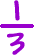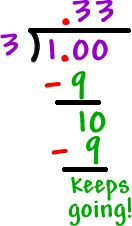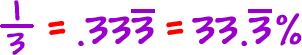So, what happens when you can't make the denominator a pretty power of 10?

Well, we just use long division and do a little rounding.  Let's just make our answers go to one decimal point.

Let's try one:

 Convertto a percent:

First, we use long division to convert the fraction to a decimal...Just like we thought, it's giving a repeating decimal:So, we can give our answer asOr we can round off and approximate the answer asNotice that I used a wavy equals for "approximately equal!"